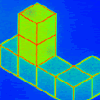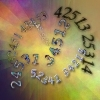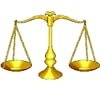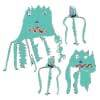# Search by Topic

#### Resources tagged with Place value similar to Subtraction Surprise:

Filter by: Content type:
Age range:
Challenge level:

### There are 73 results

Broad Topics > Numbers and the Number System > Place value### Subtraction Surprise

##### Age 7 to 14 Challenge Level:

Try out some calculations. Are you surprised by the results?### Digit Sum

##### Age 11 to 14 Challenge Level:

What is the sum of all the digits in all the integers from one to one million?### Method in Multiplying Madness?

##### Age 7 to 14 Challenge Level:

Watch our videos of multiplication methods that you may not have met before. Can you make sense of them?### What an Odd Fact(or)

##### Age 11 to 14 Challenge Level:

Can you show that 1^99 + 2^99 + 3^99 + 4^99 + 5^99 is divisible by 5?### Arrange the Digits

##### Age 11 to 14 Challenge Level:

Can you arrange the digits 1,2,3,4,5,6,7,8,9 into three 3-digit numbers such that their total is close to 1500?### Basically

##### Age 11 to 14 Challenge Level:

The number 3723(in base 10) is written as 123 in another base. What is that base?### Just Repeat

##### Age 11 to 14 Challenge Level:

Think of any three-digit number. Repeat the digits. The 6-digit number that you end up with is divisible by 91. Is this a coincidence?### Skeleton

##### Age 11 to 14 Challenge Level:

Amazing as it may seem the three fives remaining in the following `skeleton' are sufficient to reconstruct the entire long division sum.### Double Digit

##### Age 11 to 14 Challenge Level:

Choose two digits and arrange them to make two double-digit numbers. Now add your double-digit numbers. Now add your single digit numbers. Divide your double-digit answer by your single-digit answer. . . .### What Do You Need?

##### Age 7 to 11 Challenge Level:

Four of these clues are needed to find the chosen number on this grid and four are true but do nothing to help in finding the number. Can you sort out the clues and find the number?### Pupils' Recording or Pupils Recording

##### Age 5 to 14

This article, written for teachers, looks at the different kinds of recordings encountered in Primary Mathematics lessons and the importance of not jumping to conclusions!### X Marks the Spot

##### Age 11 to 14 Challenge Level:

When the number x 1 x x x is multiplied by 417 this gives the answer 9 x x x 0 5 7. Find the missing digits, each of which is represented by an "x" .### Being Collaborative - Primary Number

##### Age 5 to 11 Challenge Level:

Number problems at primary level to work on with others.### Permute It

##### Age 11 to 14 Challenge Level:

Take the numbers 1, 2, 3, 4 and 5 and imagine them written down in every possible order to give 5 digit numbers. Find the sum of the resulting numbers.### Which Scripts?

##### Age 7 to 11 Challenge Level:

There are six numbers written in five different scripts. Can you sort out which is which?### Song Book

##### Age 7 to 11 Challenge Level:

A school song book contains 700 songs. The numbers of the songs are displayed by combining special small single-digit cards. What is the minimum number of small cards that is needed?### Even Up

##### Age 11 to 14 Challenge Level:

Consider all of the five digit numbers which we can form using only the digits 2, 4, 6 and 8. If these numbers are arranged in ascending order, what is the 512th number?### Balance Power

##### Age 11 to 18 Challenge Level:

Using balancing scales what is the least number of weights needed to weigh all integer masses from 1 to 1000? Placing some of the weights in the same pan as the object how many are needed?### Eleven

##### Age 11 to 14 Challenge Level:

Replace each letter with a digit to make this addition correct.### A Story about Absolutely Nothing

##### Age 7 to 18

This article for the young and old talks about the origins of our number system and the important role zero has to play in it.### Three Times Seven

##### Age 11 to 14 Challenge Level:

A three digit number abc is always divisible by 7 when 2a+3b+c is divisible by 7. Why?### Six Times Five

##### Age 11 to 14 Challenge Level:

How many six digit numbers are there which DO NOT contain a 5?### Which Is Quicker?

##### Age 7 to 11 Challenge Level:

Which is quicker, counting up to 30 in ones or counting up to 300 in tens? Why?### Back to the Planet of Vuvv

##### Age 11 to 14 Challenge Level:

There are two forms of counting on Vuvv - Zios count in base 3 and Zepts count in base 7. One day four of these creatures, two Zios and two Zepts, sat on the summit of a hill to count the legs of. . . .### Not a Polite Question

##### Age 11 to 14 Challenge Level:

When asked how old she was, the teacher replied: My age in years is not prime but odd and when reversed and added to my age you have a perfect square...### Diagonal Sums

##### Age 7 to 11 Challenge Level:

In this 100 square, look at the green square which contains the numbers 2, 3, 12 and 13. What is the sum of the numbers that are diagonally opposite each other? What do you notice?### Being Resilient - Primary Number

##### Age 5 to 11 Challenge Level:

Number problems at primary level that may require resilience.##### Age 11 to 14 Challenge Level:

Powers of numbers behave in surprising ways. Take a look at some of these and try to explain why they are true.### Tis Unique

##### Age 11 to 14 Challenge Level:

This addition sum uses all ten digits 0, 1, 2...9 exactly once. Find the sum and show that the one you give is the only possibility.### Seven Up

##### Age 11 to 14 Challenge Level:

The number 27 is special because it is three times the sum of its digits 27 = 3 (2 + 7). Find some two digit numbers that are SEVEN times the sum of their digits (seven-up numbers)?### Repeaters

##### Age 11 to 14 Challenge Level:

Choose any 3 digits and make a 6 digit number by repeating the 3 digits in the same order (e.g. 594594). Explain why whatever digits you choose the number will always be divisible by 7, 11 and 13.### Becky's Number Plumber

##### Age 7 to 11 Challenge Level:

Becky created a number plumber which multiplies by 5 and subtracts 4. What do you notice about the numbers that it produces? Can you explain your findings?### Quick Times

##### Age 11 to 14 Challenge Level:

32 x 38 = 30 x 40 + 2 x 8; 34 x 36 = 30 x 40 + 4 x 6; 56 x 54 = 50 x 60 + 6 x 4; 73 x 77 = 70 x 80 + 3 x 7 Verify and generalise if possible.### Being Curious - Primary Number

##### Age 5 to 11 Challenge Level:

Number problems for inquiring primary learners.##### Age 5 to 11 Challenge Level:

Who said that adding couldn't be fun?### Multiply Multiples 3

##### Age 7 to 11 Challenge Level:

Have a go at balancing this equation. Can you find different ways of doing it?### Trebling

##### Age 7 to 11 Challenge Level:

Can you replace the letters with numbers? Is there only one solution in each case?### Football Sum

##### Age 11 to 14 Challenge Level:

Find the values of the nine letters in the sum: FOOT + BALL = GAME### Multiply Multiples 1

##### Age 7 to 11 Challenge Level:

Can you complete this calculation by filling in the missing numbers? In how many different ways can you do it?### The Thousands Game

##### Age 7 to 11 Challenge Level:

Each child in Class 3 took four numbers out of the bag. Who had made the highest even number?### Being Resourceful - Primary Number

##### Age 5 to 11 Challenge Level:

Number problems at primary level that require careful consideration.### Cayley

##### Age 11 to 14 Challenge Level:

The letters in the following addition sum represent the digits 1 ... 9. If A=3 and D=2, what number is represented by "CAYLEY"?### Always a Multiple?

##### Age 11 to 14 Challenge Level:

Think of a two digit number, reverse the digits, and add the numbers together. Something special happens...### One Million to Seven

##### Age 7 to 11 Challenge Level:

Start by putting one million (1 000 000) into the display of your calculator. Can you reduce this to 7 using just the 7 key and add, subtract, multiply, divide and equals as many times as you like?### Multiply Multiples 2

##### Age 7 to 11 Challenge Level:

Can you work out some different ways to balance this equation?### Number Detective

##### Age 5 to 11 Challenge Level:

Follow the clues to find the mystery number.### Legs Eleven

##### Age 11 to 14 Challenge Level:

Take any four digit number. Move the first digit to the end and move the rest along. Now add your two numbers. Did you get a multiple of 11?### All the Digits

##### Age 7 to 11 Challenge Level:

This multiplication uses each of the digits 0 - 9 once and once only. Using the information given, can you replace the stars in the calculation with figures?### Two and Two

##### Age 11 to 14 Challenge Level:

How many solutions can you find to this sum? Each of the different letters stands for a different number.### Oddly

##### Age 7 to 11 Challenge Level:

Find the sum of all three-digit numbers each of whose digits is odd.